# Primal-Dual Hybrid Gradient Algorithm (PDHG)¶

This page introduces the mathematics behind the Primal-Dual Hybrid Gradient Algorithm. For an applied point of view, please see the user’s guide to this method.

## The general problem¶

The Primal-Dual Hybrid Gradient Algorithm (PDHG) algorithm, as studied in [CP2011a], is a first order method for non-smooth convex optimization problems with known saddle-point structure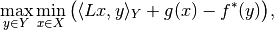where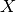and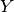are Hilbert spaces with inner product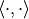and norm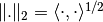,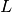is a continuous linear operator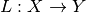,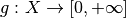andare proper, convex and lower semi-continuous functionals, and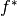is the convex (or Fenchel) conjugate of f, (see convex conjugate).

The saddle-point problem is a primal-dual formulation of the primal minimization problem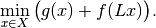The corresponding dual maximization problem is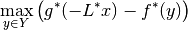with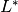being the adjoint of the operator.

## The algorithm¶

PDHG basically consists in alternating a gradient-like ascent in the dual variable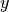and a gradient-like descent in the primal variable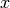. Additionally, an over-relaxation in the primal variable is performed.

### Initialization¶

Choose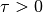,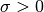,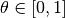,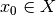,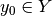,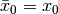### Iteration¶

For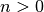update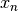,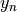, and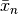as follows: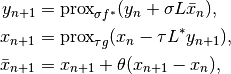Here,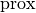stands for proximal operator.

### Step sizes¶

A simple choice of step size parameters is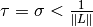, since the requirement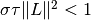guarantees convergence of the algorithm. Of course, this does not imply that this choice is anywhere near optimal, but it can serve as a good starting point.

### Acceleration¶

If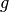oris uniformly convex, convergence can be accelerated using variable step sizes as follows:

Replace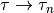,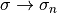, and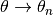and choose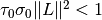and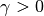. After the update of the primal variable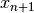and before the update of the relaxation variable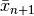use the following update scheme for relaxation and step size parameters: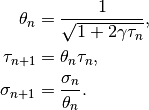Instead of choosing step size parameters, preconditioning techniques can be employed, see [CP2011b]. In this case the steps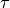and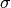are replaced by symmetric and positive definite matrices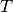and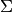, respectively, and convergence holds for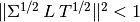.

For more on proximal operators and algorithms see [PB2014]. The implementation of PDHG in ODL is along the lines of [Sid+2012].